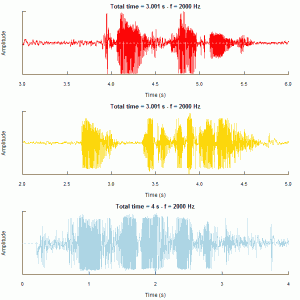# Mandelbrot Set

1.ggplot2的图形，循环迭代较多，运行确实很慢。

 1 2 3 4 5 6 7 8 9 10 11 12 13 14 15 16 17 18 19 20 21 22 23 24 25 26 27 28 29 30 31 32 33 34 35 36 37 38 39  library(ggplot2) max_iter = 25 cl = colours() step = seq(-2, 0.8, by = 0.005) points = array(0, dim = c(length(step)^2, 3)) t = 0 for (a in step) { for (b in step + 0.6) { x = 0 y = 0 n = 0 dist = 0 while (n < max_iter & dist < 4) { n = n + 1 newx = a + x^2 - y^2 newy = b + 2 * x * y dist = newx^2 + newy^2 x = newx y = newy }   if (dist < 4) { color = 24 } else { color = n * floor(length(cl)/max_iter) }   t = t + 1 points[t, ] = c(a, b, color) } } df = as.data.frame(points) ggplot(data = df, aes(V1, V2, color = cl[V3])) + geom_point(size = 0.5) + opts(panel.background = theme_blank(), panel.grid.major = theme_blank(), panel.grid.minor = theme_blank(), axis.ticks = theme_blank(), axis.text.x = theme_blank(), axis.text.y = theme_blank(), axis.title.x = theme_blank(), axis.title.y = theme_blank(), legend.position = "none")2. 动态看图形变化，此转于此处，代码简洁易理解。不过代码做出来的图与原网页展示的图形不同，颜色怎么调都没有他们的漂亮，主要是迭代次数还不够，也不够精细，直接看原链接的吧，搭配颜色是个细致活，伤脑细胞。

# 爱的声音1 2 3 4 5 6 7 8 9 10 11 12  library(seewave) library(tuneR) woaini = readMP3("我爱你.mp3") woaini1=resamp(woaini, g = 2000) muqinjie = readMP3("母亲节快乐.mp3") muqinjie1 = resamp(muqinjie, g = 2000) iloveyou = readMP3("i_love_you.mp3") iloveyou1 = resamp(iloveyou, g = 2000) op = par(mfrow = c(3,1),mar = c(4.5,4,2,2)) oscillo(woaini1, f = 2000, from = 3, to = 6,cexlab = 0.75,colwave = "red",title = "妈-我爱你") oscillo(muqinjie1, f = 2000, from = 2, to = 5,cexlab = 0.75,colwave="gold",title = "妈-母亲节快乐") oscillo(iloveyou1, f = 2000, from = 0, to = 4,cexlab = 0.75,colwave="lightblue",title = "i love you so much")• 最上是我用家乡话（信阳罗山话）说的：“妈，我爱你！”（罗山话中，“你”发音类似于“恩”，故振幅小）
• 中间一幅依然用家乡话：“妈，母亲节快乐！”（录得不好，貌似有杂音）
• 最下面的那副是英文：“I love you so much！”

# 提高Monte Carlo模拟定积分计算的精度

• 平均值方法是根据弱大数定律证明得来的。构造如此

均方误差

而由$Schwartz$不等式知

,从中便可知 $MC$ 法具有半阶收敛性，且收敛阶不依赖问题的维数，这样在计算高维积分的时候，比较优越。但是收敛速度还是很慢的，是一个比较坏的收敛速度。因此在有其他高效率、高精度的确定型算法时，就不要采用$MC$法，那是在没有更好的算法时更好的抉择，比如超高维积分。
• 由均方误差可知，要提高 $MC$ 法的精确度，那么久必须得增大$N$，减小$VAR(f)$，也就是说，要增加样本量，同时改善抽样方法的选择，这时便有很多方法选择，书中提到了四种方法，我就谈谈我的理解和试验。
1. 分层抽样。核心就是将原来的 $[0,1]$ 区间 $M$ 等分后在各个小区间上分别再以均匀分布抽样 $N/M$ 个点，然后以

理论证明知这样方差减少，具体证明式子较多就不再写，否则就是一本教材的翻版了。
模拟$\int_{0}^{1}exp(-x^2/2)/\sqrt{2pi}dx$,具体实验结果如下

 1 2 3 4 5 6 7  M<-100 N<-1000 A=matrix(as.numeric(gl(M,N/M))-1,ncol=N/M,byrow=T) B=replicate(M,runif(N/M)) x=1/M*t(B)+A/M y=exp(-x^2/2)/sqrt(2*pi) I=mean(y)

结果为0.3413287，可知只取了1000个样本点的情况下，精确度已经十分好了，而一般的均值模拟
达到此精确度则需要$10^7$个点。

2. 对偶变量法。
这是一种特殊的针对于定义域具有一定对称性的函数性质的技巧。基于这样的命题:如果$f(x)$是单调的，则$Cov(f(X),f(1-X))\leq0$,这里$X\sim U[0,1]$。则有

,
$EI_N=I(f)$,
经过证明知 $Var(I_N)\leq \frac{1}{2N}Var(f)$，方差显然减少。

但是这种方法只是对于定义域有一定对称性的函数，如果对一种函数先验了解不多，而且对称性不知，则运用会出现失误，所以具有一定局限性。
模拟$\int_{0}^{1}exp(-x^2/2)/\sqrt{2pi}dx$的代码如下：

 1 2 3  a=runif(10^6) y=exp(-a^2/2)/sqrt(2*pi)+exp(-(1-a)^2/2)/sqrt(2*pi) b=sum(y)/(2*10^6)

结果为 0.3413487，精确度也得到了提高。

3. 重要性抽样：核心就是构造一个新的积分

其中$p(x)$$[0,1]$的概率密度，$\int_{0}^{1}p(x)dx=1$,$p(x)\geq 0$。这样得到类似于最初最简单的那种均值法的新的形式积分近似$I(f)\approx 1/N\sum_{i=1}^{1}f(Y_i)/p(Y_i)$$Y_i$为遵循p(y)的独立同分布随机变量(i.i.d),而之所以这样构造，可以根据这幅图来理解，$\int_{0}^{a}+\int_{b}^{1}f(x)dx$$I(f)$ 很少比例(即图像的边缘部分)，尽可能的不让随机点去计算这些值，这样的“拟合”积分较好（面积）,否则很多的随机点在 $[a,b]$ 外，为计算小比例的东西做贡献，这样必定精度较差，所以我们要期望尽可能的值出现在峰值处。我们称其为“重要性”抽样。理论分析，

,

.
只要选取适当的 $p(y)$ 就可以使方差减少。
当然不可能是$Var(f/p)=0$，这在图的模拟便可知，$p(y)$不可能与$f(x)$相合的同时，还有$\int_{0}^{1}p(x)dx=1$。但是$p$难以选择，需要对问题先验有了一定的了解程度才能适当选取。比如对于正态分布求$\int_{0}^{1}exp(-x^2/2)/\sqrt(2pi)dx$，我不太好选$p$，就没有做相关的模拟了。
4. 控制变量法。
核心也是构造，数学式子$I_N(f)=i/N\sum_{i=1}^{N}(f-g)(X_i)+I(g)$.
需要$I(g)$已知，但是这是同重要性抽样一样，都不知道，也需要对问题先验有了一定的了解程度，才能好的选择，使$I(g)$已知,并且有$Var(f-g)\leq Var(f)$。相关模拟我也没有再做